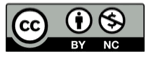抛物线抛物线的定义二次项系数的影响一次项系数的影响单位抛物线

$$y = x^2$$

$$y' = a (y + b)\\x' = a (x + b)$$反射性质Orthoptic 性质